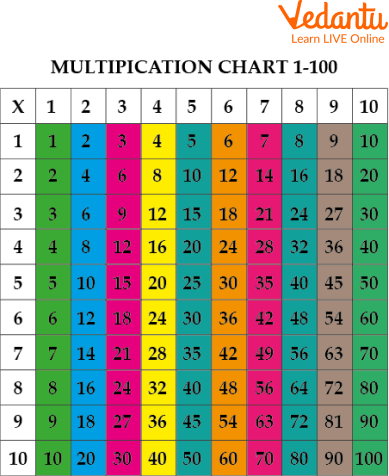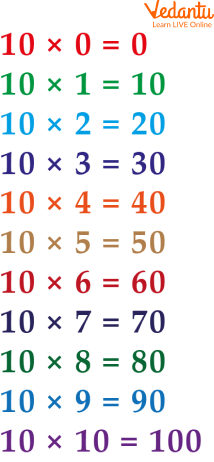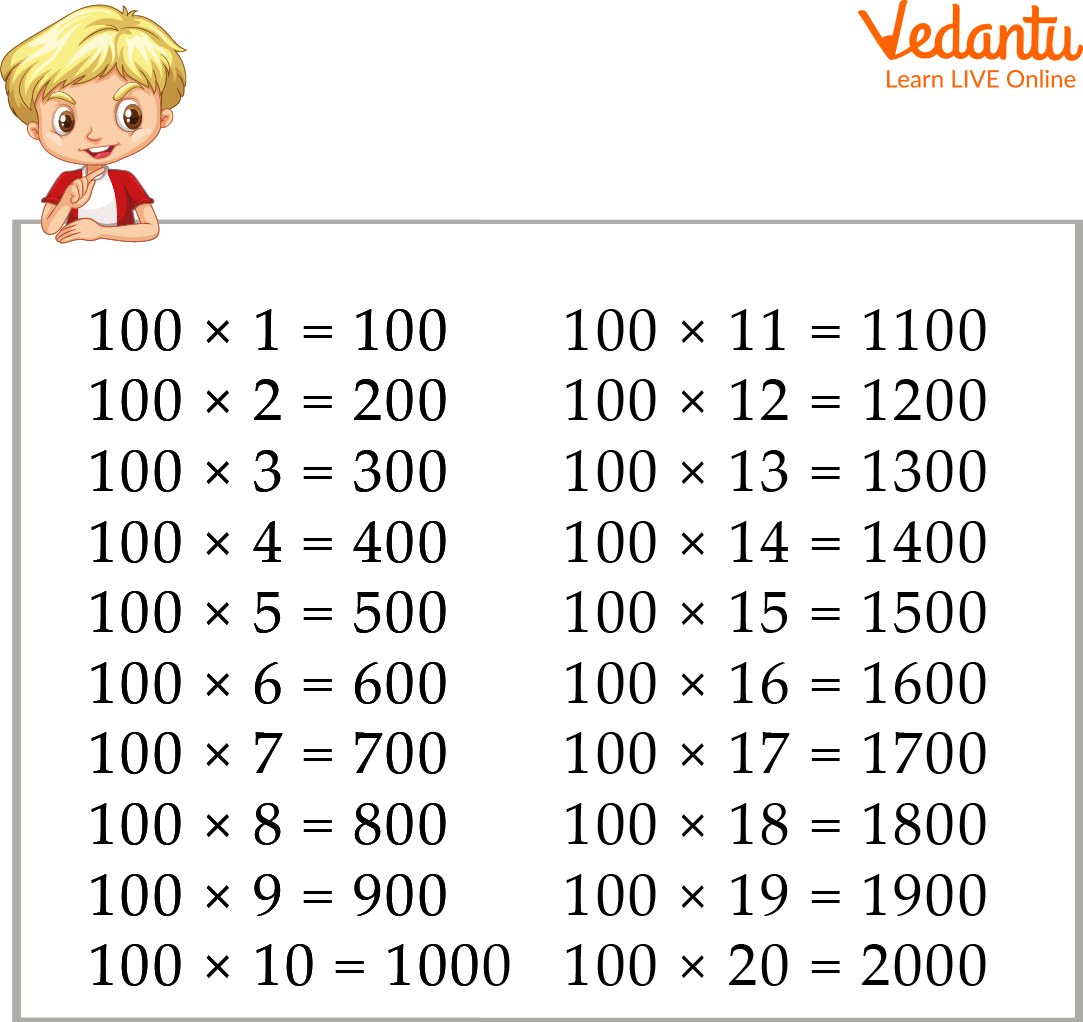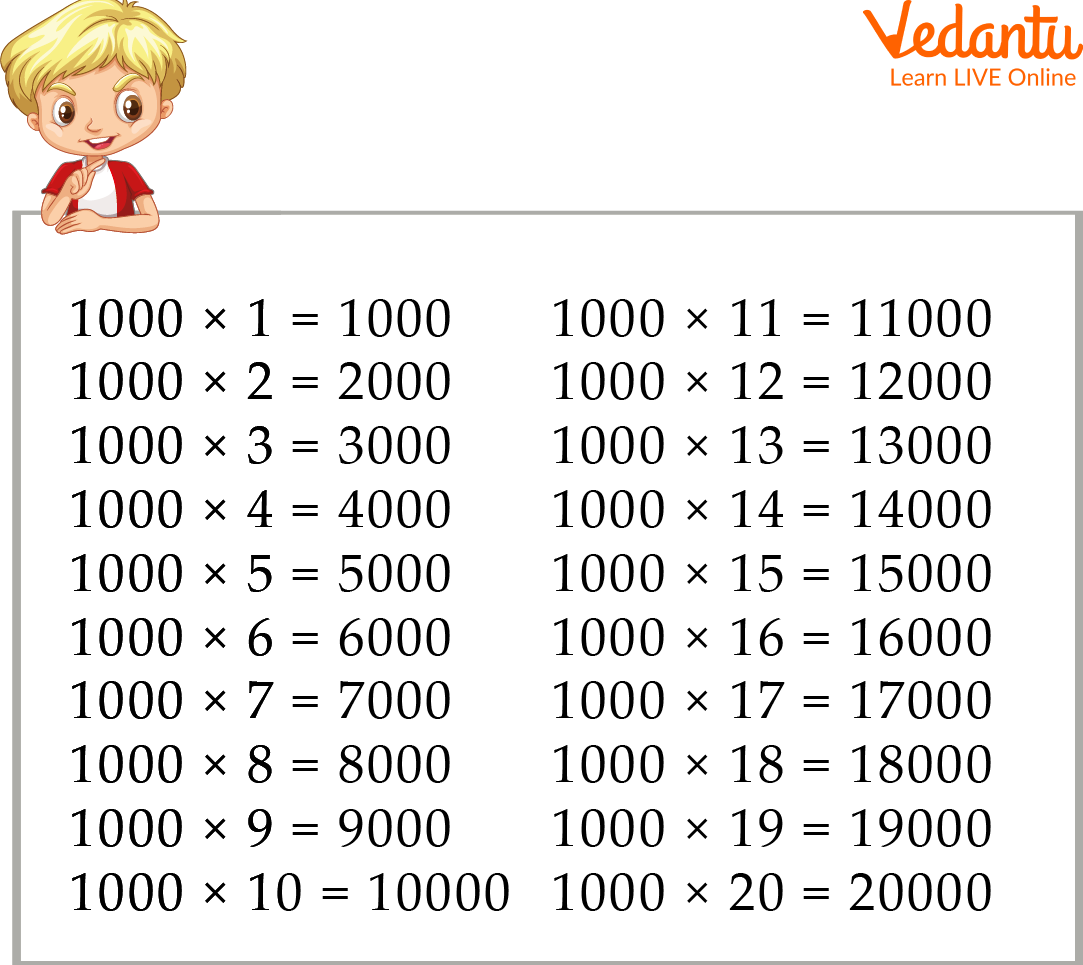Courses
Courses for Kids
Free study material
Offline Centres
More

# Multiplication by 10, 100 and 1000Last updated date: 05th Dec 2023
Total views: 98.4k
Views today: 0.98k## A Brief Explanation of Multiplication by 10, 100 and 1000

Mathematical multiplication by 10s, 100s, and 1000s is a significant topic. It is utilized in a number of mathematical operations, including multiplication, division, and percentage. There are two alternative ways to accomplish it. The long method and the short method. The short method is easier to do, but it takes more time. The long method takes less time, but it's harder to do than the short method.

The process of multiplying by 10, 100 multiplication and 1000 involves finding the product of the first number multiplied by 10, then the next number multiplied by 100, and then the last number multiplied by 1000.

In this topic, we will mainly discuss things related to multiplication by 10, 100 and 1000. Apart from this, in this topic, we will also look at worksheets and examples related to them, which will help us better understand this topic.

## Multiplication Chart with One to Hundred Table

Here one to hundred tables are given, which help to improve this topic:Multiplication Chart

## Multiplication Table of 10

Here are the multiplication tables of 10, which are like thisTable of 10

## Multiplication Table of 100

Here are the multiplication tables of 100, which are like thisTable of 100

## Multiplication Table of 1000

Here are the multiplication tables of 1000, which are like thisTable of 1000

The result of a multiplication by 100 is the integer with two zeros to its right. The result of a multiplication by 1000 is the number with three zeros to the right of the original integer. The result of a 10x calculation is the number with 1 zero to the right of the original.

## Multiply by 10, 100 and 1000 Example

Here is an example for multiplying 10 by 100 and 1000, which is as follows:

Example: Multiply 1.3 with 10

Ans: Here, as per the question, we must multiply 1.3 by 10.

So, when a whole number is multiplied by ten, the multiplicand is followed by one zero.

So on multiplying a decimal by 10, we shift the decimal point to the right by one place, i.e. $1.3 \times 10 = 13.0$

Example- Multiply 3.62 with 100

Ans: Here, as per the question, we must multiply 3.62 by 100.

So as we know, when a whole number is multiplied by 100, the multiplicand is followed by two zeros.

So on multiplying a decimal by 100, we shift the decimal point to the right by two places i.e. $3.62 \times 100 = 3.6200 = 362$

Example- Multiply 56.3 with 1000

Ans: Here, as per the question, we must multiply 5.63 by 1000.

So as we know, when a whole number is multiplied by 1000, the multiplicand is followed by three zeros.

So on multiplying a decimal by 1000, we shift the decimal point to the right by three places i.e. $56.3 \times 1000 = 5.63000 = 563.0$

## Multiply by 10, 100 and 1000 Worksheet

Here is a worksheet for multiplying 10 by 100 and 1000, which is as follows:

Q 1. Multiply 47 × 10

Ans: 470

Q 2. Multiply 57.25 × 100

Ans: 5725

Q 3. Multiply 125.056 × 1000

Ans: 125,056

Q 4. Multiply 29 × 100

Ans: 2900

Q 5. Multiply 25.63 × 10

Ans: 2,563

## Summary

The significance of multiplication by 10s, 100s, and 1000s in math was covered in this article. Multiplication by 10, 100 and 1000 is important because it is a way to represent large numbers in a more manageable form. It is also an important concept to learn because it can be used in many other areas of mathematics. In conclusion, the 10, 100, and 1000 multiplication methods are very simple and easy to use. They can be used to find the product of two numbers.

## FAQs on Multiplication by 10, 100 and 1000

1. What makes multiplying by 10 different from multiplying by 100?

Multiplying by 10 is the same as adding 1 to the number. For example, if you multiply by 10, then 1 becomes 11 and 2 becomes 12. Multiplying by 100 is the same as multiplying by 10. If you multiply by 100, then 1 becomes 101 and 2 becomes 102. The difference between multiplying by 10 and multiplying by 100 is the number of digits in the result. For example, 10 x 10 = 100, but 100 x 100 = 10000.

2. What are the steps to multiplying by 10, 100, and 1000?

The steps to multiply by 10, 100, and 1000 are the same.

Step 1: Multiply the number by 10

Step 2: Multiply the number by 100

Step 3: Multiply the number by 1000

3. What is the difference between 100 and 1000?

The difference between 100 and 1000 is the number of times you multiply by ten. 1000 equals 10x10x10x10x10x10x10. 100 equals 10.

4. How can you multiply two numbers together in maths?

The multiplication operation is an easy one to understand. It is a process that requires two numbers and the result of multiplication. The answer to this question depends on what you are multiplying together. For example, multiplying two whole numbers together will result in a product that is a whole number. Multiplication of fractions will result in a product that is a fraction. When multiplying decimals, the answer will be another decimal number.